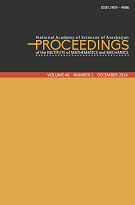﻿ Online First — Proc. Inst. Math. Mech.P R O C E E D I N G S
of the
Institute of Mathematics and Mechanics

 ISSN 2409-4986 (Print) ISSN 2409-4994 (Online)
 Home Editorial Board For Authors Publication Ethics Online First Volumes
 Converse theorem of the approximation theory of functions in Morrey-Smirnov classes related to the derivatives of functions by Sadulla Z. Jafarov Scalability of \$G\$-frames by diagonal operators by Ahmad Ahmadi and Asghar Rahimi On the error of approximation by RBF neural networks with two hidden nodes by Arzu M-B. Babayev and Ibrahim K. Maharov Nonlinear wave equations with nonlinear transmission acoustic condition by Sevda E. Isayeva On integration of the loaded Korteweg-de Vries equation in the class of rapidly decreasing functions by Aknazar B. Hasanov and Umid A. Hoitmetov On an inverse problem for a parabolic equation in a domain with moving boundaries by Adalat Ya. Akhundov and Arasta Sh. Habibova A study of harmonic sections of tangent bundles with vertically rescaled Berger-type deformed Sasaki metric by Abderrahim Zagane Calderón-Zygmund operators with kernels of Dini's type and their multilinear commutators on generalized variable exponent Morrey spaces by Vagif S. Guliyev and Afag F. Ismayilova The well-posedness of the mixed problem for one system of thermoelasticity with a singular coefficient by Akbar B. Aliyev and Gunay R. Gadirova Solvability of a boundary value problem for a second order elliptic differential-operator equation with a complex parameter by Bahram A. Aliev, Vugar Z. Kerimov and Yakov S. Yakubov
 © 2018 Institute of Mathematics and Mechanics